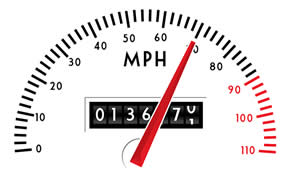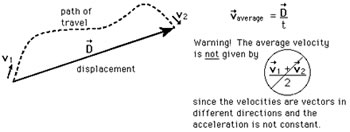# Difference between Average Velocity and Average Speed

##### Key difference: Average Velocity defines the total amount of distance from the starting point, which is also known as displacement, divided by the amount of time passed. Whereas, the Average Speed denotes the total distance traveled over a certain amount of time.Average Velocity and Average Speed are two terms that are often confused. The confusion mainly arises due to the fact that the terms denote something quite similar. Average Velocity defines the total amount of distance from the starting point, which is also known as displacement, divided by the amount of time passed. Whereas, the Average Speed denotes the total distance traveled over a certain amount of time.

They sound exactly the same; however, there are certain differences between the two. The main difference is the fact that speed is a scalar quantity, which means that it has no directions. Velocity, on the other hand, is a vector quantity, which means that it has directions. Another difference between the two is the fact that average speed calculates the average distance traveled, whereas, average velocity calculates the average displacement.

While, these differences may seem minor, they have a major impact. Let’s consider an example:

Mary drives to work every morning. However, she stops to buy coffee on the way there. So, Mary drives to the coffee shop, and then drives to work. Imagine now, that the distance between Mary’s home and Mary’s office is 18 miles. However, the distance between Mary’s home and the coffee shop is 8 miles. The distance between the coffee shop and Mary’s office is 13 miles.So every morning, Mary drives 8 miles to the coffee shop, then another 13 miles to the office and it takes approximately one and a half hour to get to work. So, the total distance that Mary drives is 8 + 13 = 21 miles. The total distance divided by total time is average speed. Hence, Mary’s average speed is 21 miles/1.5 hours = 14 miles/hour.

However, average velocity calculates the average displacement. Mary’s office is 18 miles away from her home. So, Mary shifted 18 miles from her home to her office. Hence, her average velocity is the total displacement divided by total time, i.e. 18 miles / 1.5 hours = 12 miles/hour.

Comparison between Average Velocity and Average Speed:

 Average Velocity Average Speed Definition according to Wikipedia The average velocity of an object moving through a displacement during a time interval. The average speed of an object in an interval of time is the distance traveled by the object divided by the duration of the interval. Definition The average displacement traveled per hour The average distance traveled per hour; the distance traveled divided by the time taken Formula Average Velocity = Total displacement/time interval Average Speed = Distance covered/time interval Units m/sec, cm/sec, ft/sec, km/h, miles/hr, etc. m/sec, cm/sec, ft/sec, km/h, miles/hr, mph, etc. Example If a particle is moving along the x axis and is located at 10 meters at 2 seconds and at 12 meters at 4 seconds, then the average velocity in that particular time interval would be = (12-10)/(4-2 )= 5 m/s If a distance of 80 kilometers is driven in 1 hour, the average speed is 80 kilometers per hour. Likewise, if 320 kilometers are traveled in 4 hours, the average speed is also 80 kilometers per hour. Purpose When there are displacements corresponding to the different time taken, then we need to determine the average velocity. When a particular distance has been covered over time, and one needs to figure out the

Image Courtesy: forbesconsulting.com, hyperphysics.phy-astr.gsu.edu

## Add new comment

### Plain text

CAPTCHA
This question is for testing whether or not you are a human visitor and to prevent automated spam submissions.